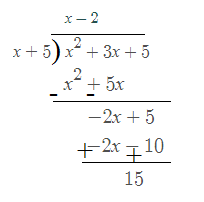# Slant Asymptote Formula

When a polynomial has a numerator higher than the denominator, then a slant asymptote occurs. Slant asymptote can also be referred to an oblique. To find the oblique, we need to divide the numerator to the denominator using synthetic division method or long division. The numerator being stronger, “pulls” the graph far from the x-axis or other fixed y value. The distance of the curve is so close that they approach if extended until infinity.

### Formula For Slant Asymptote

If for example we have a function : f(x), then the slant asymptote will be in the form:

$$\begin{array}{l}\large f(x)=\frac{ax^{n}+bx^{n-1}+…}{cx^{n-1}+….}\end{array}$$

### Solved Example

Question: Find the Slant Asymptote for the function: y =

$$\begin{array}{l}\frac{x^2 + 3x + 5}{x + 5}\end{array}$$

Solution:

Given function is y =

$$\begin{array}{l}\frac{x^2 + 3x + 5}{x + 5}\end{array}$$
. Here the degree of numerator is more than that of denominator by 1. Hence the given function is a slant asymptote.The slant asymptote for the function y =

$$\begin{array}{l}\frac{x^2 + 3x + 5}{x + 5}\end{array}$$
is (x – 2).